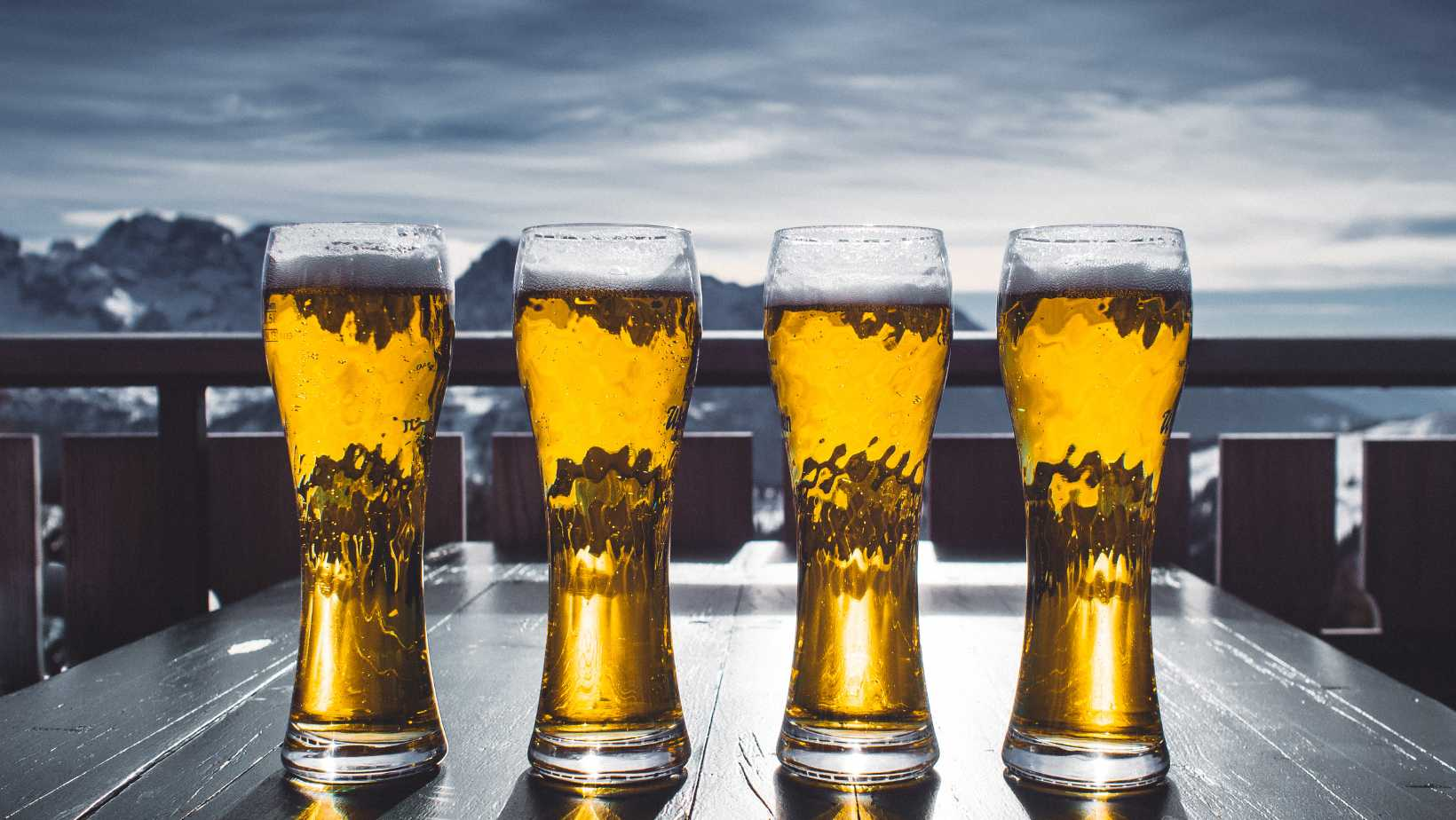# How Many ML Are in a Pint? – Exploring The Conversion Between Milliliters And PintsWhen it comes to converting measurements, knowing how many milliliters are in a pint is essential. So, let’s dive right into the answer! There are 473.176 milliliters in a US pint, while in an imperial pint (used in countries like the UK), there are approximately 568.261 milliliters.

Understanding this conversion can be quite useful in various situations. For instance, if you’re following a recipe that uses metric measurements and it calls for a pint of milk or water, you’ll now know that you need around 473 milliliters (or about half a liter) to achieve the desired result.

Moreover, having this knowledge can come in handy when comparing beverage sizes at restaurants or bars. If you prefer metric units and want to know how much liquid you’re getting with a pint-sized drink, simply keep in mind that it’s equivalent to roughly 473 milliliters.

Now that we’ve answered the question of how many ml are in a pint, you’ll be able to confidently navigate conversions and understand the volume represented by this common unit of measurement. Cheers to expanding your knowledge!

## How Many Ml Are in a Pint

### Converting Pints to Milliliters: A Simple Guide

If you’ve ever found yourself in a situation where you needed to convert pints to milliliters, you’re not alone. Understanding the conversion between these two units of measurement is essential for various tasks, such as cooking, bartending, or even scientific experiments. Let’s dive into a simple guide on how many ml are in a pint.

To convert pints to milliliters, we need to know the conversion factor. In this case, it’s important to note that one pint is equal to approximately 473.18 milliliters (ml). This means that if you have a certain number of pints and want to know the equivalent volume in milliliters, all you need to do is multiply by 473.18.

For example:

• 1 pint = 473.18 ml
• 2 pints = 946.35 ml
• 3 pints = 1419.53 ml

### The Conversion Formula: Pints to Milliliters Explained

Now that we know the conversion factor between pints and milliliters, let’s break down the formula:

Milliliters (ml) = Number of Pints × 473.18

When converting from pints to milliliters, simply take the number of pints and multiply it by 473.18 using this straightforward formula.

### Common Uses for Pint-to-Milliliter Conversions

Understanding how many ml are in a pint can come in handy across various scenarios:

1. Cooking: Recipes often list ingredients with measurements in different units like cups or pints while some kitchen tools provide measurements in milliliters.
2. Mixology: Bartenders frequently use both imperial and metric units when crafting cocktails and understanding conversions ensures accuracy.
3. Lab Experiments: In scientific experiments, precise measurements are crucial, and being able to convert between pints and milliliters is essential for accuracy.
4. Beverage Packaging: Some beverages may be labeled in pints or milliliters depending on the country of origin or target market. Understanding the conversion helps consumers make informed choices.

Knowing how to convert pints to milliliters opens up a world of possibilities in various fields. Whether you’re a home cook, bartender, scientist, or consumer, having this knowledge gives you the flexibility to work with different units of measurement seamlessly.

Remember that when it comes to conversions, precision matters. Always double-check your calculations and use the appropriate tools like measuring cups or digital scales for accurate results.

Now that we’ve explored how many ml are in a pint and learned about converting between these units, let’s move on to other informative sections of this article!

## Converting Pints to Milliliters

When it comes to converting pints to milliliters, it’s important to have the right conversion factor in mind. The conversion factor for this particular conversion is 1 pint equals approximately 473.18 milliliters.

To make it easier for you to understand, let me break it down into a few steps:

1. Start with the given number of pints you want to convert.
2. Multiply that number by the conversion factor of 473.18 milliliters per pint.
3. The result will be the equivalent volume in milliliters.

Let’s look at an example:

If I have 2 pints and I want to know how many milliliters they are, I’ll follow these steps:

2 pints * 473.18 milliliters/pint = 946.36 milliliters

So, 2 pints is equal to approximately 946.36 milliliters.

Here’s a handy table showing some common conversions from pints to milliliters:

 Pints Milliliters 1 473.18 2 946.36 3 1419.54 … …

It’s worth noting that these conversions are approximate due to rounding off decimal places.

Now that you know how many milliliters are in a pint and how to convert between the two units, you can confidently tackle any measurement or recipe that requires this conversion.

Remember, practice makes perfect! So go ahead and put your newfound knowledge into action whenever needed.

Happy converting!

Amanda is the proud owner and head cook of her very own restaurant. She loves nothing more than experimenting with new recipes in the kitchen, and her food is always a big hit with customers. Amanda takes great pride in her work, and she always puts her heart into everything she does. She's a hard-working woman who has made it on her own, and she's an inspiration to all who know her.